Chapter 3: Rigid Body Basics

# 3.6 Examples

Here are examples from Chapter 3 to help you understand these concepts better. These were taken from the real world and supplied by FSDE students in Summer 2021. If you’d like to submit your own examples, please send them to the author eosgood@upei.ca.

# Example 3.6.1: Reaction Forces, Submitted by Andrew Williamson

1. Problem

A family is sitting watching TV on their couch. The couch is 5 m long and weighs 120 N. The child is sat 1 m away from one end and has a mass of 30 kg. The mother is sat 0.5 m away from the child and has a mass of 60 kg. The father is 3 m away from the mother and has a mass of 70 kg.

a) Draw a free-body diagram of the couch

b) Calculate the reaction force on each of the two legs.

Assume the couch is supported by two rollers.

2. Draw

Sketch: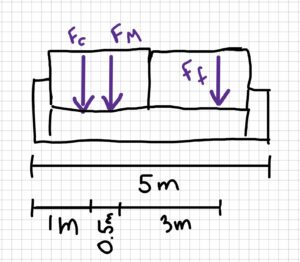3. Knowns and Unknowns

Knowns:

• g = 9.81 m/s2
• mc = 30 kg
• mM = 60 kg
• mf = 70 kg
• Fg = 120 N
• rc = 1 m
• rM = 1.5 m
• rf = 4.5 m
• rB = 5 m
• rg = 2.5 m

Unknowns: NA, NB

4. Approach

Use equilibrium equations

5. Analysis

Part a: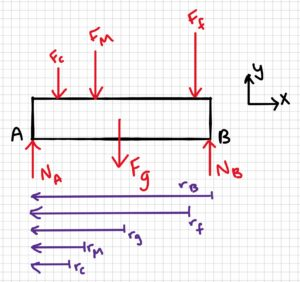Part b:

$$\sum M_B=0=N_B\cdot r_B-F_c\cdot r_c-F_M\cdot r_M-F_f\cdot r_f-F_g\cdot r_g\\\\N_B(5m)=(30kg\cdot 9.81m/s^2)(1m)+(60kg\cdot 9.81m/s^2)(1.5m)\\+(70kg\cdot 9.81m/s^2)(4.5m)+(120N)(2.5m)$$$$\\N_B(5m)=294.3Nm+882.9Nm +3090.15Nm+300Nm\\\\N_B(5m)=4567.35N m\\\\N_B\frac{4567.35Nm}{5m}\\\\N_B=913.47N\\\\N_B=913N$$

$$\sum F_y=0=N_A+N_B-F_C-F_M-F_f-F_g\\\\N_A=F_C+F_M+F_f+F_g-N_B\\\\N_A=(30kg\cdot 9.81m/s^2)+(60kg\cdot 9.81m/s^2)\\+(70kg\cdot 9.81m/s^2)+120N-913.47N$$$$\\N_A=294.3N+588.6N+686.7N+120N-913.47N$$$$\\N_A=776.13N\\\\\\\underline{N_A=776N}$$

6. Review

It is interesting that NB is larger than NA, because the weight of the mother and child combined (80 kg) is larger than that of the father (70 kg). However, when you sum the moments at point B instead of A, you get the same answer. The distance between the reaction forces and the nearest forces is important, as well as the magnitude of the forces themselves. The distance between A and Fc is 1 m, while the distance between B and Ff is only 0.5 m.

Additionally, it makes sense that both NA and NB are positive, i.e. are in the positive y direction.

# Example 3.6.2: Couples, Submitted by Kirsty MacLellan

1. Problem

A water valve is opened by a wheel with a diameter of 10 inches. It takes 7.5 lb of force to open the valve. What is the moment it takes to open the valve?

Real-life scenario:

2. Draw

Sketch: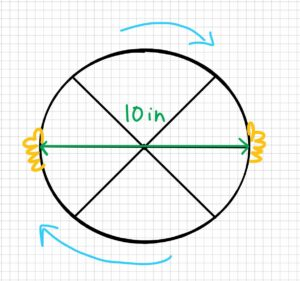Free-body diagram: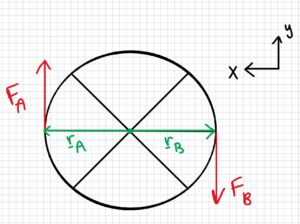3. Knowns and Unknowns

Known:

d = 10 in

F = 7.5 lb

Unknown: M

4. Approach

Determine the moment by finding the cross product of rA and FA, then rB and FB, then add.

5. Analysis

$$r=\frac{d}{2}\\r=\frac{10in}{2}\\r=5in\\5in\times\frac{1ft}{12in}=0.42ft$$

Find rA, FA, rB, and FB in vector form:

$$\underline{r}_A= \begin{bmatrix} 0.42 \\ 0 \end{bmatrix}ft\:\; \underline{F}_A=\begin{bmatrix} 0 \\ 7.5 \end{bmatrix}lb \\\underline{r}_B=\begin{bmatrix} -0.42 \\ 0 \end{bmatrix}ft\:\; \underline{F}_B=\begin{bmatrix} 0 \\ -7.5 \end{bmatrix}lb$$

Find MA:

$$\underline{M}_A=\underline{r}_A\times \underline{F}_A=\begin{bmatrix} \underline{\hat{i}} & \underline{\hat{j}} & \underline{\hat{k}} \\ 0.42 & 0 & 0 \\ 0 & 7.5 & 0 \end{bmatrix}$$ $$\underline{M}_A=\hat{i} \begin{bmatrix} 0 & 0 \\ 7.5 & 0 \end{bmatrix} -\underline{\hat{j}} \begin{bmatrix} 0.42 & 0 \\ 0 & 0 \end{bmatrix}+\underline{\hat{k}} \begin{bmatrix} 0.42 & 0 \\ 0 & 7.5 \end{bmatrix}\\\underline{M}_A=(\underline{\hat{i}}(0)-\underline{\hat{j}}(0)+\underline{\hat{k}}(o.42\cdot 7.5-0\cdot 0))ft\cdot lb\\\underline{M}_A=3.15\underline{\hat{k}} ft\cdot lb$$

Find MB:

$$\underline{M}_B=\underline{r}_B\times \underline{F}_B=\begin{bmatrix} \underline{\hat{i}} & \underline{\hat{j}} & \underline{\hat{k}} \\ -0.42 & 0 & 0 \\ 0 & -7.5 & 0 \end{bmatrix}$$$$\underline{M}_B=\hat{i} \begin{bmatrix} 0 & 0 \\ -7.5 & 0 \end{bmatrix} -\underline{\hat{j}} \begin{bmatrix} -0.42 & 0 \\ 0 & 0 \end{bmatrix}+\underline{\hat{k}} \begin{bmatrix} -0.42 & 0 \\ 0 & -7.5 \end{bmatrix}\\\underline{M}_B=(\underline{\hat{i}}(0)-\hat{j}(0)+\underline{\hat{k}}(-0.42\cdot -7.5-0\cdot 0))ft\cdot lb\\\underline{M}_B=3.15\underline{\hat{k}} ft\cdot lb$$

Add MA and MB to get M:

$$\underline{M}=\underline{M}_A+\underline{M}_B\\\underline{M}=3.15ft\cdot lb+3.15ft\cdot lb\\\underline{M}=6.3\underline{\hat{k}}ft\cdot lb$$

6. Review

This answer makes sense because there is only moment acting in the k direction.

Note: we could have come to the same answer using the formula M = F*d, which would have been faster.

$$M=f\cdot d\\M=7.5lb\cdot 10in(\frac{1ft}{12in})\\M=7.5lb\cdot \frac{5}{6}ft\\\\M=6.25ft\cdot lb$$

This answer is slightly more accurate, because we didn’t round when converting between inches and feet (in the original solution, we rounded 0.416667 to 0.42).

# Example 3.6.3: Distributed Load, Submitted by Luciana Davila

1. Problem

A shelf on the wall is 1.5 meters away from the floor. The shelf has a length of 100 cm. A person starts putting different objects on it to create a distributed load. The load created a curve described by:

w = 4x4 +2 N/m.

Calculate the resultant force and how far it is acting from the wall.

2. Draw

Sketch: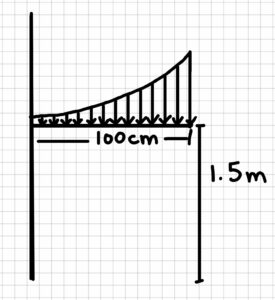Free-body diagram: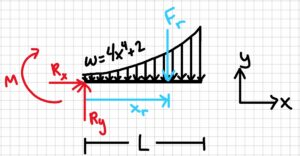3. Knowns and Unknowns

Knowns:

• w = 4x4 + 2 N/m
• L = 100 cm
• xmin = 0
• xmax = 1

Unknowns: xr, Fr

4. Approach

Use distributed load equations:

$$F_r=\int^{xmax}_{xmin} wdx\\X_r=\frac{\int^{xmax}_{xmin} x*w(x)*dx}{\int^{xmax}_{xmin}wdx}$$

5. Analysis

Solve for Fr:

$$F_r=\int^1_0 (4x^4+2)dx\;\; N\\F_r=(\frac{4x^5}{5}+2x)\vert^1_0\;\;N\\F_r=(\frac{4}{5}+2)N\\F_r=2.8N$$

Solve for xr:

$$X_r=\frac{(\int^1_0x(4x^4+2)dx)N/m}{\int^1_0(4x^4+2)dx)N}\\X_r=\frac{\int^1_0(4x^5+2x)dxN/m}{2.8N}\\X_r=\frac{(\frac{4x^6}{6}+\frac{2x^2}{2})\vert^1_0N/m}{2.8N}\\X_r=\frac{(\frac{2}{3}+1)N/m}{2.8N}\\X_r=0.59m$$

6. Review

The function shows an increasing curve on the interval, so it makes sense that the resultant force would be applied closer to the right end of the beam than the left end.